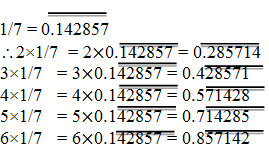Newbie

# You know that 1/7 = 0.142857. Can you predict what the decimal expansions of 2/7, 3/7, 4/7, 5/7, 6/7 are, without actually doing the long division? If so, how? [Hint: Study the remainders while finding the value of 1/7 carefully.] Q.2

• 1

What is the solution of this important question of the chapter number system . How i solve the exercise 1.3 question number 2 in a easiest way , find the best solution of this question also find the simplest solution of this question.You know that 1/7 = 0.142857. Can you predict what the decimal expansions of 2/7, 3/7, 4/7, 5/7, 6/7 are, without actually doing the long division? If so, how? [Hint: Study the remainders while finding the value of 1/7 carefully.]

Share